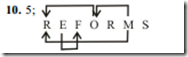Monday, 31 October 2016

1. Suresh ranks seventh from the top and thirty eighth from the bottom in a class. How many students are there in the class?
1) 40
2) 45
3) 42
4) 44
5) None of these

2. Among J, K, L, M and N, each having a different salary, J gets less than M. L gets more than M but less than N. Who among them gets the highest salary?
1) N
2) Either N or K
3) L
4) Can’t be determined
5) None of these

3. What should come in place of question mark (?) in the following  series  based  on  the  English  alphabetical series?
CAT FDW IGZ ?
1) LDH
2) LJC
3) LIJ
4) KTC
5) None of these

4. Four of the following five are alike in a certain way and so  form  a  group.  Which  is  the  one  that  does  not belong to that group?
1) Tiger
2) Ounce
3) Jackal
4) Leopard
5) Panther

5. N is sister of W. I is brother of W. U is father of N and G is mother of I. How is G related to W?
1) Daughter
2) Son
3) Mother
5) None of these

Directions (Q. 6-9): Study the following information carefully and answer the given questions:
A man has five luggage's V, W, X, Y and Z, each having a  different  weights. V  weighs  twice  as  much  as W.  W weighs four-and-a-half times as much as X. X weighs half as much as Y. Y weighs half as much as Z, and Z weighs less than V but more than X.

6. Which of the following luggage's is the heaviest?
1) Z
2) W
3) V
4) Can’t be determined
5) None of these

7. What is the weighs of Z if X weighs 1 kg?
1) 4 kg
2) 2.5 kg
3) 2 kg
4) Can’t be determined
5) None of these

8. How many luggage's are lighter than W?
1) Two
2) Three
3) Four
4) Can’t be determined
5) None of these

9. What is the weight of V if X weighs 1 kg?
1) 5 kg
2) 8 kg
3) 7.5 kg
4) 9 kg
5) None of these

10. How many such pairs of letters are there in the word REFORMS each of which has as many letters between them in the word as in the English alphabet?
1) None
2) One
3) Two
4) Three
5) More than three1. 4

Explain: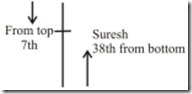The total  number of  students =  38 +  7  –  1 =  44

2. 2;  N  >  L  >  M  >  J  of  these,  N  gets  the highest  salary.  But  we  don’t  have  any information  about  K.  It  is  possible  even  K gets the  highest  salary. Hence,  either  K or  N

3. 2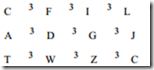4.3; Except Jackal, all others are cat category animals.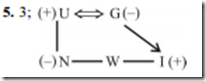(6-9):
We can assume the  weight of luggage X as 1 kg
The weight of luggage Y is 1 × 2 = 2 kg
The weight of luggage Z is 2 × 2 = 4 kg
The weight  of luggage W is 1 × 4.5 = 4.5 kg
The weight of luggage V = 4.5 × 2 = 9 kg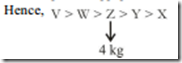6. 3

7. 1

8. 2

9.4